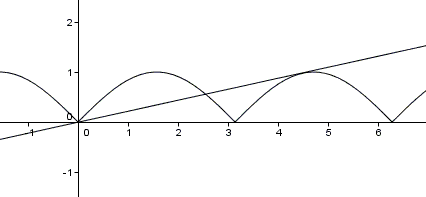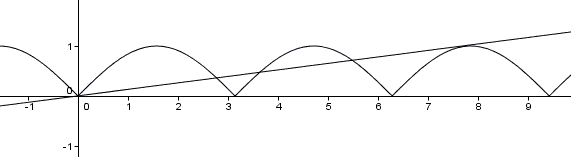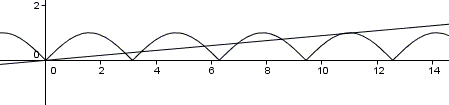# On the Intersection of kx and |sin(x)|

### Problem

It's known that the curve $y=|\sin (x)|$ intercepts the line $y=kx$ ($k\gt 0)$ at exactly three points, the maximum $x$ coordinate of those points being $\alpha.$ Prove that

$\displaystyle\frac{\cos\alpha}{\sin\alpha +\sin 3\alpha}=\frac{1+\alpha^2}{4\alpha}.$

### Hint

What can be said about the third intersection of $y=kx$ with $y=|\sin (x)|?$### Solution

The straight line $y=kx$ intersects the first arc of $y=|\sin (x)|$ in two points, leaving a single intersection for the second arc. A straight line and a convex arc (that, in this case, could be thought as closed) have a single intersection only when the two are tangent to each other.The abscissa of the tangency point belongs to the interval $(\pi, 3\pi/2),$ where $\sin(x)\lt 0.$ In this interval, the given function equals $y=-\sin(x),$ with the derivative being $y'=-\cos(x).$ Thus, the slope $k$ of the line is, on one hand, $-\cos\alpha$ and, on the other hand, $\displaystyle -\frac{\sin\alpha}{\alpha},$ which leads to $\alpha =\tan\alpha.$

Now, use some basic trigonometry:

\begin{align}\displaystyle \frac{\cos\alpha}{\sin\alpha +\sin 3\alpha}&=\frac{\cos\alpha}{2\sin 2\alpha \cos \alpha} =\frac{1}{4\sin\alpha \cos\alpha} \\ &=\frac{\sin^{2}\alpha+\cos^{2}\alpha}{4\sin\alpha \cos\alpha} =\frac{1+\tan^{2}\alpha}{4\tan\alpha} \\ &=\frac{1+\alpha^2}{4\alpha} \end{align}

### Generalize!

What if the number of intersections is $5$?And what if it is $7$?### Acknowledgment

This is a problem from the 2009 Chinese Mathematics Competition [Xiong Bin, p 13].

### References

1. Xiong Bin, Lee Peng Yee, Mathematical Olympiads in China (2009-2010). Problems and Solutions, World Scientific, 2013### Trigonometry